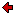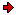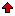Journal of Technical
Physics

A QUARTERLY JOURNALPrevious issue vol. 46, no. 1 (2005) vol. 46, no. 2 (2005)Next issuevol. 46, no. 3 (2005)

### Contents of issue 2, vol. 46

1. M.A. Ezzat, M. Zakaria, A.S. El-Karamani: A general model of generalized thermoelasticity with temperature-dependent modulus of elasticity
2. S. Piekarski: On the thermodiffusion equation for electrically charged matter
3. A. Rewiński, T. Łukaszewicz, J. Żmija: Li+ ionic conductivity calculations of solid electrolytes on polymeric basis
4. J. Mazur, J. Spalińska-Mazur, J. Ryrych: Anomalous movement resistance in a spinning gyroscope
5. A. Szczepański: The status of quantum information transfer at the individual level

M.A. Ezzat, M. Zakaria, A.S. El-Karamani: A general model of generalized thermoelasticity with temperature-dependent modulus of elasticity
A new formula of generalized thermoelasticity equations for isotropic media is established. The present model does apply to generalizations, the Lord-Shulman theory with one relaxation time and the Green-Lindsay theory with two relaxation times, as well as to the coupled theory. The normal mode analysis is used to obtain the exact expressions for the temperature distribution, thermal stresses, and the displacement components. The resulting formulation is applied to three different concrete problems. The first one deals with a thick plate subject to heating on parts of the upper and lower surfaces of the plate; the second one concerns the case of a heated punch moving across the surface of a semi-infinite thermoelastic half-space subject to appropriate boundary conditions; and the third problem deals with a plate with thermo-insulated surfaces subjected to time-dependent compression. Numerical results are given and illustrated graphically for the problem considered. A comparison was made with the results obtained in the case of temperature-independent modulus of elasticity in each theory.

ContentsS. Piekarski: On the thermodiffusion equation for electrically charged matter
In this paper, we propose the thermodiffusion equation for electrically charged matter. It is a generalisation of the modified Fick law, which has been introduced in the paper: S. Piekarski, "On the modified Fick law and its potential applications" (J. Tech. Phys., 44, 2, 125-131, 2003). In the modified Fick law there exists a potential force acting on the diffusing matter which is described as the gradient of the potential U(x). Now we replace U(x) by U(x) - qφ(x,t) where φ is the electrostatic potential, while q is the electric charge of a diffusing carrier. We write the corresponding field equations and consider the electromotive force induced by the temperature gradient. It is easy to compute this force under the assumption that the system is quasineutral. However, it seems that a more realistic model of the electromotive force induced by the temperature gradient should allow a deviation from quasineutrality. Both versions are compared and it is interesting whether the resulting expressions can be verified experimentally.

ContentsA. Rewiński, T. Łukaszewicz, J. Żmija: Li+ ionic conductivity calculations of solid electrolytes on polymeric basis
This paper presents a theoretical approach to Li+ ions diffusion in polymeric solid electrolytes. The following parameters were calculated and then compared with the experimental results: the mobility of lithium ions, diffusion coefficient and recombination probability. Ionic conductivity of the electrolyte was also calculated.

ContentsJ. Mazur, J. Spalińska-Mazur, J. Ryrych: Anomalous movement resistance in a spinning gyroscope
A symmetric harnessed gyroscope accelerated to a given spinning frequency takes different time periods to stop, depending on the direction of previous spins. For repeated alternating, anticlockwise and clockwise spinning, the rotation period in both directions significantly increases, which is not the case when the gyroscope is repeatedly rotated in the same direction. Using the measurements it was observed, that the time of gyroscope's rotation was significantly lengthened or shortened, what indicates that it either increased or decreased the movement resistance of the gyroscope. The presented experimental results suggest the existence of anomalous movement resistance and demonstrate that a fixed spinning gyroscope displays unusual history-dependent movement resistance effects. The effect is real, large, reproducible and does not follow from experimental errors.
Key words: spinning gyroscope, movement resistance, clockwise rotation, anticlockwise rotation.

ContentsA. Szczepański: The status of quantum information transfer at the individual level
Two feasible energy-correlation experiments with twin photons from parametric down-conversion are proposed. A new method to determine the lower bound of quantum information transfer speed is proposed in the first experiment. Classical information transfer exclusively by means of the quantum channel is the expected result of the second experiment.

ContentsReprints of the full papers may be obtained from their authors. Contact Editorial Office in case you need the address of the respective author.
 Home Editors Scope Abstracts For authors Subscription